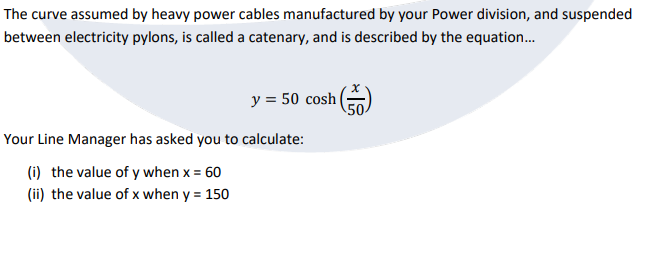Home / Expert Answers / Electrical Engineering / the-curve-assumed-by-heavy-power-cables-manufactured-by-your-power-division-and-suspended-between-pa378

# (Solved): The curve assumed by heavy power cables manufactured by your Power division, and suspended between ...The curve assumed by heavy power cables manufactured by your Power division, and suspended between electricity pylons, is called a catenary, and is described by the equation... Your Line Manager has asked you to calculate: (i) the value of when (ii) the value of when

We have an Answer from Expert

To calculate the values requested, we can simply substitute the given values into the equation for the catenary curve.

(i) To find the value of y when x = 60

Substituting x = 60 into the equation

Using the definition of the hyperbolic cosine function,

Calculating this expression will give us the value of y.

Let's simplify the expression step by step
First, we can simplify the exponentials

Now, substitute these values back into the equation
We have an Answer from Expert